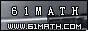Today is . WELCOME: huohai3983/44663  Home | Reg Login | All user | setHomePage | BookmarkHome | Math Test Online | Addition | Subtraction | Multiplication | Division | Time | Worksheet(JPG/PDF) | CAI | Flash Games |Recommendation articleArticle searchHome--maths--Second Grade--Adding Hundreds

How to add three-digit numbers (for example 600 + 500).

• Place one number above the other so the hundreds' place digits, the tens' place digits and ones' place digits are lined up. Draw a line under the bottom number.
```600
500
```
• Add the two ones' place digits. (0 + 0 = 0).
```600
500
0
```
• Add the numbers in the tens' place column (0 + 0 = 0) and place the answer below the line and to the left of the ones' place sum.
``` 600
500
00```
• Add the numbers in the hundreds' place column (6 + 5 = 11) and place the answer below the line and to the left of the tens' place sum.
```  600
500
1100```

#### Find the Sum of the Numbers.

 =

2006-10-02 20:12:54
Viewed 3220 times CloseGreatmathsites